# On line

On line p: x = 4 + t, y = 3 + 2t, t is R, find point C, which has the same distance from points A [1,2] and B [-1,0].

x =  2
y =  -1

### Step-by-step explanation: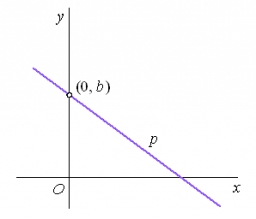Did you find an error or inaccuracy? Feel free to write us. Thank you!Tips to related online calculators
Line slope calculator is helpful for basic calculations in analytic geometry. The coordinates of two points in the plane calculate slope, normal and parametric line equation(s), slope, directional angle, direction vector, the length of the segment, intersections of the coordinate axes, etc.
Looking for help with calculating roots of a quadratic equation?
Do you have a linear equation or system of equations and looking for its solution? Or do you have a quadratic equation?
Pythagorean theorem is the base for the right triangle calculator.

#### You need to know the following knowledge to solve this word math problem:

We encourage you to watch this tutorial video on this math problem:

## Related math problems and questions:

• On a lineOn a line p : 3 x - 4 y - 3 = 0, determine the point C equidistant from points A[4, 4] and B[7, 1].
• Sphere equationObtain the equation of sphere its centre on the line 3x+2z=0=4x-5y and passes through the points (0,-2,-4) and (2,-1,1).
• Calculate 6Calculate the distance of a point A[0, 2] from a line passing through points B[9, 5] and C[1, -1].
• Distance problemA=(x, x) B=(1,4) Distance AB=√5, find x;
• Find the 5Find the equation of the circle with center at (1,20), which touches the line 8x+5y-19=0
• Equation of circle 2Find the equation of a circle that touches the axis of y at a distance of 4 from the origin and cuts off an intercept of length 6 on the axis x.
• Distance between 2 pointsFind the distance between the points (7, -9), (-1, -9)
• Right triangle from axesA line segment has its ends on the coordinate axes and forms with them a triangle of area equal to 36 square units. The segment passes through the point ( 5,2). What is the slope of the line segment?
• Center of line segmentCalculate the distance of the point X [1,3] from the center of the line segment x = 2-6t, y = 1-4t ; t is from interval <0,1>.
• Find the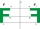Find the image A´ of point A [1,2] in axial symmetry with the axis p: x = -1 + 3t, y = -2 + t (t = are real number)
• Distance problem 2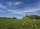A=(x,2x) B=(2x,1) Distance AB=√2, find value of x
• Construct 8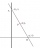Construct an analytical geometry problem where it is asked to find the vertices of a triangle ABC: the vertices of this triangle must be the points A (1,7) B (-5,1) C (5, -11). the said problem should be used the concepts of: distance from a point to a li
• Find the 3Find the distance and midpoint between A(1,2) and B(5,5).
• Curve and lineThe equation of a curve C is y=2x² -8x+9, and the equation of a line L is x+ y=3 (1) Find the x coordinates of the points of intersection of L and C. (2) Show that one of these points is also the stationary point of C?
• Distance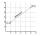Calculate distance between two points X[18; 19] and W[20; 3].
• Isosceles triangleIn an isosceles triangle ABC with base AB; A [3,4]; B [1,6] and the vertex C lies on the line 5x - 6y - 16 = 0. Calculate the coordinates of vertex C.
• Find parametersFind parameters of the circle in the plane - coordinates of center and radius: ?